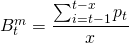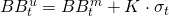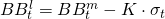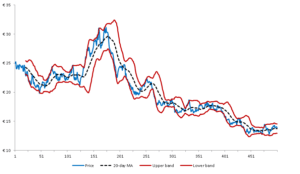# Bollinger bands

Bollinger bands give an indication by the current price of a security lies relatively compared to its past prices. This give you an indication whether a price is more or less expensive compared to the recent past. This is achieved in 2 ways: by measures a simple moving average of past prices and by measuring the price action, volatility of the security.

## Bollinger bands formula

Bollinger bands can be easily calculated using the following procedure. First calculate the middle band. This is simple a simple moving average using a look back period x. A parameter value  of 20 as look back is often found in literature.The upper Bollinger band is simple the sum of the middle band and a multiple, K, of the past standard deviation. A value for K=2 and a look back similar like the calculation of the SMA is used for the standard deviation.Likewise, the lower Bollinger band is calculated by subtracting a multiple of the past standard deviation from the middle band.